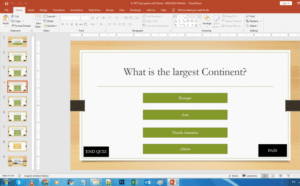# Populate A PowerPoint Quiz From Excel With VBA

Import Questions & Answers of a Microsoft PowerPoint Multiple-Choice Quiz Game from Excel with VBA.

We can automatically generate a PowerPoint Quiz Game whilst using Microsoft Excel as our database. We can type the questions and answers in pre-set columns, then using VBA in PowerPoint we can populate our Question Slides.

Our Microsoft Excel Sheet shall contain 5 colums: Question, Correct Answer; and the other 3 shall denote the Wrong Answers.

Since we know that the questions are in the ‘A’ Column, we can add a For Loop from A2 to A(x), where x refers to one addition to the total number of questions.

Once we are able to import the data from Excel, we need to replace the text of the shapes in our PowerPoint Slides.

We can first change the names of the shape via Selection Pane (as shown in the video), and then use the .TextFrame.TextRange Property to change the text of the question and answer shapes!

We run the following ImportQuestions sub-routine to populate our PowerPoint Quiz Game. We first set the location of the Excel Workbook that is already created in the same folder as the PowerPoint Quiz Game.

Our columns are preset with the data that is supposed to be present. The A Column has only the questions, the B Column has only the correct answer, the columns from C to E only have the wrong answers.

This later allows us to call to the values present in the Excel Cells of those Columns and use them to populate all the Question and Answer Shapes of the PowerPoint Quiz.``````Sub ImportQuestions()

Dim xlsWB As Object
Set xlsWB = CreateObject("Excel.Application").Workbooks.Open(ActivePresentation.Path & "\DATA.xlsx")

For i = 3 To 5
ActivePresentation.Slides(i).Shapes("TextBox 4").TextFrame.TextRange = xlsWB.Worksheets(1).Range("A" & i - 1)

ActivePresentation.Slides(i).Shapes("a1").TextFrame.TextRange = xlsWB.Worksheets(1).Range("B" & i - 1)
ActivePresentation.Slides(i).Shapes("a2").TextFrame.TextRange = xlsWB.Worksheets(1).Range("C" & i - 1)
ActivePresentation.Slides(i).Shapes("a3").TextFrame.TextRange = xlsWB.Worksheets(1).Range("D" & i - 1)
ActivePresentation.Slides(i).Shapes("a4").TextFrame.TextRange = xlsWB.Worksheets(1).Range("E" & i - 1)

Next i

xlsWB.Close

Set xlsWB = Nothing
End Sub ``````

We use a For-Loop for all the question slides. This allows us to populate all the question slides with the necessary questions and answers.

Do note that the names of the shape in PowerPoint are case-sensitive. Also, change the variable range in the for-loop based on the number of slides in your PowerPoint Quiz Game.

The biggest question now is that if we duplicate the slides and import the questions from an Excel Sheet, the answers will not be shuffled and the order and the position would remain the same. Worry not!

Send Report Card to Google Sheets, Import Questions from Excel.
Make your quiz game in 54 seconds!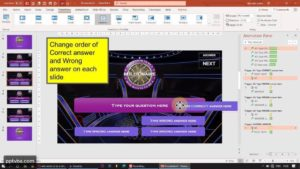## Create “Who Wants To Be A Millionaire” PowerPoint Game

Here, we will be using Microsoft PowerPoint to make an...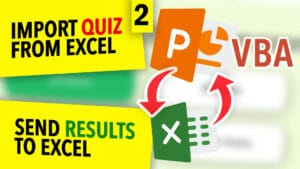## Populate A PowerPoint Quiz From Excel With VBA

Import Questions & Answers of a Microsoft PowerPoint Multiple-Choice Quiz...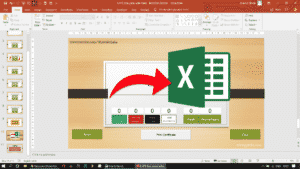## SAVE POWERPOINT QUIZ RESULTS TO EXCEL SHEET​

We will be exporting/sending the results of the PowerPoint Quiz...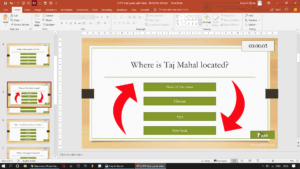## SHUFFLE QUESTIONS SLIDES & ANSWER ORDER RANDOMLY IN PPT QUIZ GAME​

We will be shuffling the order of the questions such...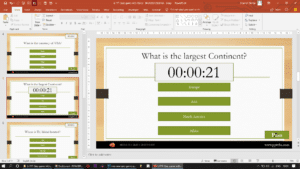## POWERPOINT QUIZ GAME WITH TIMER

As soon as the time limit is up, the student...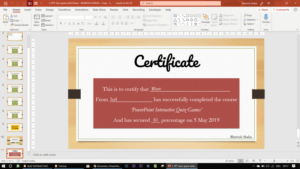## PRINT CERTIFICATE IN PPT QUIZ GAME

Collect student's name via an input field and use VBA...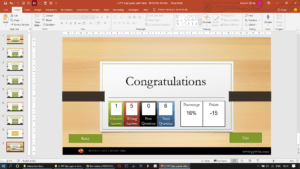## REPORT CARD AND PERCENTAGE – INTERACTIVE POWERPOINT QUIZ GAME

In this PowerPoint Quiz Game, we will calculate the number...